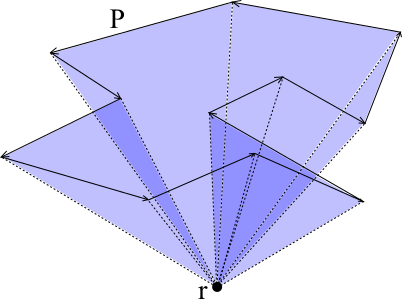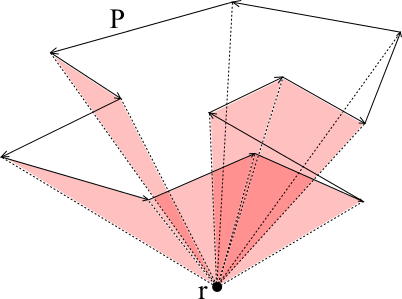Computing Optimal Polygons

Given a set of points S in the plane, it is natural to ask for the polygon that uses all these points as vertices and that is minimal or maximal with respect to covered area or length of the boundary. By distinguishing between simple and general polygons this results in 8 different problems.Eight different problems in polygon generation.

The minimum boundary simple polygon is equivalent to the Traveling Salesman Problem (TSP) and therefore NP-hard. Fekete proved that computing a simple polygon with maximum or minimum area is also NP-hard. The same also holds for general polygons with maximum or minimum area. In a recent paper of Fekete et al. NP-hardness was proven for computing non-simple polygons with minimum boundary. However, it is unknown if computing a (simple) polygon with maximum boundary is NP-hard.

When computing a polygon we work on a complete oriented graph of the point set S where the edges get some weights, e.g. the Euclidean distance between each point for optimizing the boundary of the polygons. In case we want to optimize the area of the polygon, we compute for each edge the area of the triangle that the edge forms with a reference point. If the edge has a Right-To-Left orientation relative to the reference this is the weight of the edge or otherwise we count the area negative. This way, the sum of all used edges is the area of the polygon as depicted in the figure below.Left: Building triangles. Middle: Triangles with Right-To-Left oriented edges. Right: Triangles with Left-To-Right oriented edges.

Now, with correct edge weights, we can formulate an Integer Program (IP) seen below. With (1) we ensure that the polygon will have optimized boundary or area. To get a polygon we want to have one incoming and one outgoing edge at each vertex (2). Then we need to take care of non-crossing edges (3)-(4). With these constraints we may get disjoint cycles, hence, we would not have a valid polygon. To avoid those cycles, we add the constraint (5). Because there are up to exponential many cycles, we add the cycle elimination constraints in so called separation steps.IP Formulation for generating Polygons

With this IP we can generate polygons with optimized boundary or area. To solve such an IP we use CPLEX in C++. Below you can see an instance which has different solutions for all eight problems.Top: Minimizing; Bottom: Maximizing
From left to right: Area; Boundary; Simple Area; Simple Boundary

There are still some open problems:

• Is MaxBound NP-Hard?
• Is SMaxBound NP-Hard?
• Is there a more efficient IP to solve the Problems?

Theses

No entries found.

Ist hier derzeit keine offene Arbeit zu vergeben? Oder interessiert dich das Projekt, aber es ist einfach nicht das richtige Thema für dich dabei? Dann wende dich einfach direkt an uns! Wir haben laufend Ideen für mögliche Themen in verschiedenen Bereichen, die aber vielleicht im Moment noch nicht zu einer konkreten Aufgabenbeschreibung ausgearbeitet sind. Vielleicht finden wir dann gemeinsam auch für dich eine passende und interessante Aufgabe.

HiWi Jobs

No entries found.

Project members

NameEMailPhoneRoom
Andreas Haashaas[[at]]ibr.cs.tu-bs.de+49-531-3913117
Dominik Krupkekrupke[[at]]ibr.cs.tu-bs.de+49-531-3913116315
Arne Schmidtaschmidt[[at]]ibr.cs.tu-bs.de+49-531-3913115319

Publications

• Sándor P Fekete, Andreas Haas, Michael Hemmer, Michael Hoffmann, Irina Kostitsyna, Dominik Krupke, Florian Maurer, Joseph SB Mitchell, Arne Schmidt, Christiane Schmidt and others: Computing nonsimple polygons of minimum perimeter, in International Symposium on Experimental Algorithms, Springer, pages 134-149, 2016 (fekete2016computing, BibTeX)
• Melanie Papenberg: Exact Methods for area-optimal Polygons, Masterarbeit, University of Technology Braunschweig, 2014 (Papenberg2014, BibTeX)

Further Information

You are welcome to contact the project members for further information.